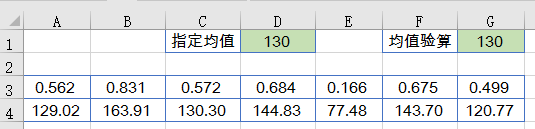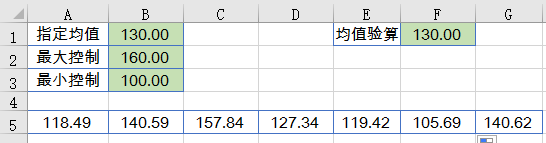# 【電腦】如何讓數據「造假」更快更真？

1、首先添加輔助行，在A3單元格輸入公式向右填充，得到7個隨機數。

=RAND()

2、在A4單元格輸入公式後向右填充，即可得到答案。

=(1+A3-AVERAGE(\$A\$3:\$G\$3))*\$D\$1=(B2-B3)*RAND()+B3

=IF(AVERAGE(\$A5:A5)<\$B\$1,(\$B\$2-\$B\$1)*RAND()+\$B1,(\$B1-\$B3)*RAND()+\$B3)

G5單元格公式為：

=7*\$B\$1-SUM(\$A5:F5)